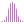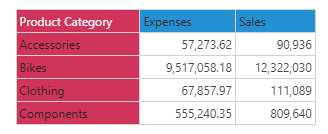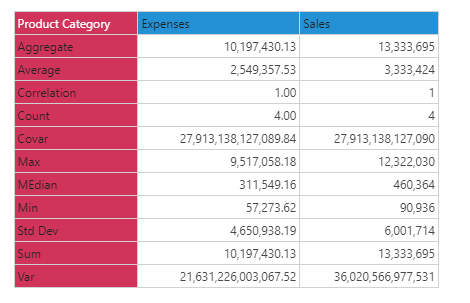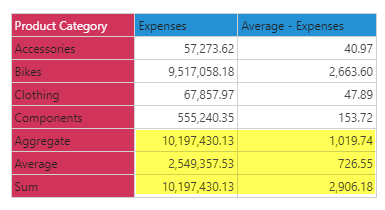#Sum (PQL)

Returns the sum of the elements in the specified list.

#### Syntax

Sum( <List> , OPTIONAL )

* Click on the function's arguments above for more details on the input values.

• List parameter should be a list or set of elements from an existing hierarchy in the model.
• The data point or tuple is optional, and describes the values used to generate the sum. If not supplied, the default measure or the query context will determine this value instead.
• For details on how to employ and use this function see the semantic calculation overview.
##### Different Function types
• This function is like the MDX sum function; it calculates a summation across a list of elements in a hierarchy or attribute.
• If, instead, you wish to change the aggregate of a numeric column in the database to sum, use this function
• To use a simple sum mathematical operation on a list of numbers, use the Common library function.

#### Examples

This example returns the sum of expenses by product category in the product table/ dimension, using the sample demo model:

Sum( {AllMembers([products].[Product Category])}, ([measures].[data Expenses]) )

Below, using the sample data of the 4 product categories we can use the above formula to calculate the total. Totaling the 4 items produces 10,179,430:#### Sum vs Aggregate

A common item confusing users is the difference between "sum" and "aggregate". When applied to additive aggregate (granular) values, there is no difference between them. As per the example below, they produce identical outcomes on the simple summation of "expenses". However, when used on a more non-additive (granular) aggregate like "Average Expenses"you get different results (2,906 vs 1,019). The sum function is a simple addition of all average values for each category (40.97+2663+47.89+153.72). Aggregate (1019) is more nuanced as shows the "average expenses" across all 4 categories, calculated at the grain. This is in contrast to the "Average" here of 726.55, which is the sum of 2906 divided by the 4 categories.

Users will need to know which value they are after when picking the function. In general, Aggregate should be used instead of Sum, because it is more often correct for most usage scenarios.See AGGREGATE for more details.